# Samacheer Kalvi 10th Maths Solutions Chapter 4 Geometry Ex 4.2

## Tamilnadu Samacheer Kalvi 10th Maths Solutions Chapter 4 Geometry Ex 4.2

Question 1.
In ΔABC, D and E are points on the sides AB and AC respectively such that DE || BC
(i) If $$\frac{\mathbf{A D}}{\mathbf{D B}}=\frac{3}{4}$$ and AC = 15 cm find AE.
(ii) If AD = 8x – 7, DB = 5x – 3, AE = 4x – 3 and EC = 3x – 1, find the value of x.
Solution: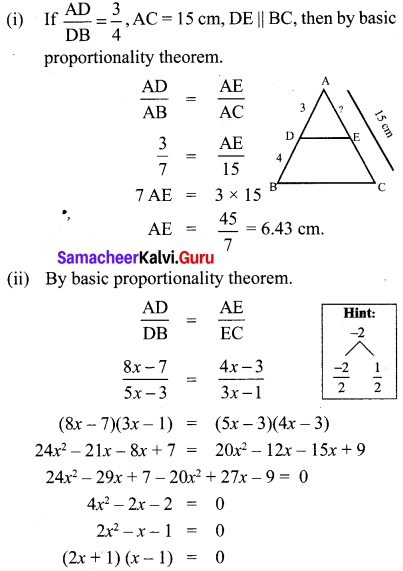x = 1, $$\frac{-1}{2}$$ ⇒ x = 1

Question 2.
ABCD is a trapezium in which AB || DC and P,Q are points on AD and BC respectively, such that PQ || DC if PD = 18 cm, BQ = 35 cm and QC = 15 cm, find AD.
Solution:
Any line parallel to the parallel sides of a trapezium dives the non-parallel sides proportionally.
∴ By thales theorem, In ΔACD, we have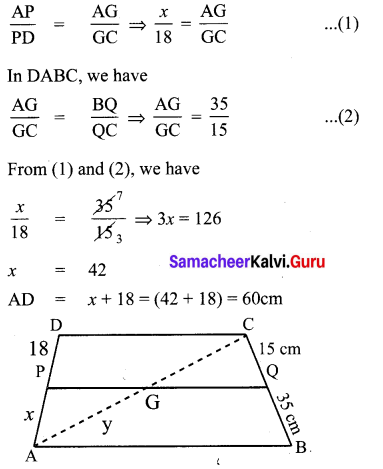Question 3.
In ΔABC, D and E are points on the sides AB and AC respectively. For each of the following cases show that DE || BC
(i) AB = 12 cm, AD = 8 cm, AE = 12 cm and AC = 18 cm.
(ii) AB = 5.6 cm, AD = 1.4 cm, AC = 7.2 cm and AE = 1.8cm.
Solution: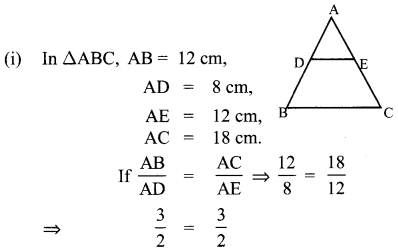∴ It is satisfied
∴ DE||BC

(ii) AB = 5.6 cm,
AC = 7.2 cm,
AE = 1.8 cm.
If $$\frac{\mathrm{AB}}{\mathrm{AD}}=\frac{\mathrm{AC}}{\mathrm{AE}}$$ is satisfied then BC || DE
$$\frac{5.6}{1.4}=\frac{7.2}{1.8}$$
5.6 × 1.8 = 1.4 × 7.2
10.08 = 10.08
L.H.S = R.H.S
∴ It is satisfied
∴ DE||BCQuestion 4.
In fig. if PQ || BC and PR ||CD prove that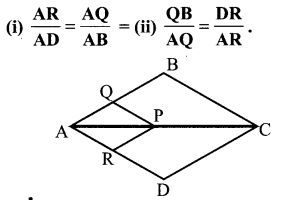Solution:
In the figure PQ || BC, PR ||CD.Question 5.
Rhombus PQRB is inscribed in ∆ABC such that ∠B is one of its angle. P, Q and R lie on AB, AC and BC respectively. If AB = 12 cm and BC = 6 cm, find the sides PQ, RB of the rhombus.
Solution:
In ∆CRQ and ∆CBA
∠CRQ = ∠CBA (as RQ || AB)
∠CQR = ∠CAB (as RQ || AB)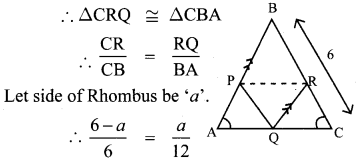⇒ 72 – 12a = 6a
⇒ 18a = 72
a = 4
Side of rhombus PQ, RB = 4 cm, 4 cm.Question 6.
In trapezium ABCD, AB || DC, E and F are points on non-parallel sides AD and BC respectively, such that EF || AB . Show that $$\frac{{A E}}{{E D}}=\frac{{B F}}{{F C}}$$
Solution:Question 7.
In figure DE || BC and CD || EF . Prove that AD2 = AB × AF.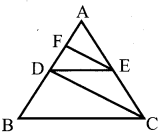Solution:Question 8.
In a ∆ABC, AD is the bisector of ∠A meeting side BC at D, if AB = 10 cm, AC = 14 cm and BC = 6 cm, find BD and DC.
Solution: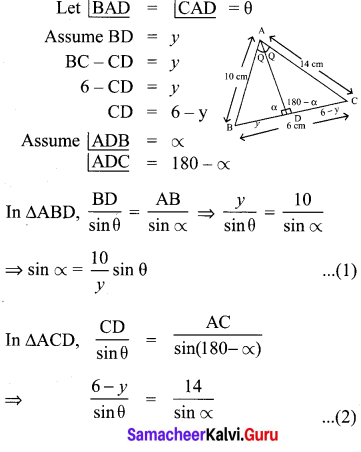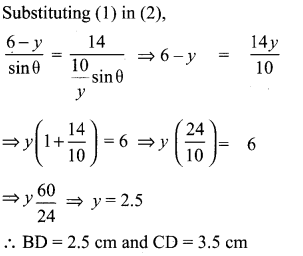Question 9.
Check whether AD is bisector of ∠A of ∆ABC in each of the following
(i) AB = 5 cm, AC = 10 cm, BD = 1.5 cm and CD = 3.5 cm.
(ii) AB = 4 cm, AC = 6 cm, BD = 1.6 cm and CD = 2.4 cm.
Solution:
AB = 5 cm,
AC = 10 cm,
BD = 1.5 cm,
CD = 3.5 cm,Question 10.
In figure ∠QPC = 90°, PS is its bisector. If ST⊥PR, prove that ST × (PQ + PR) = PQ × PR.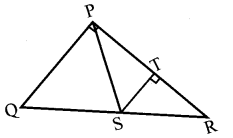Solution: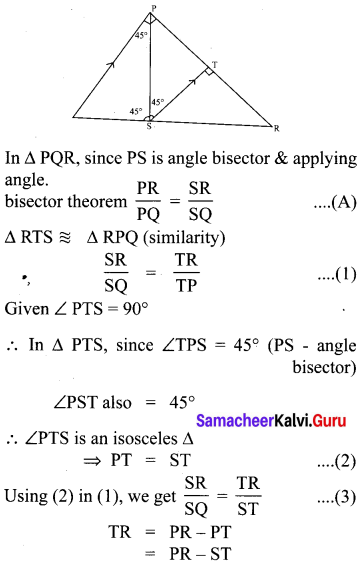Question 11.
ABCD is a quadrilateral in which AB = AD, the bisector of ∠BAC and ∠CAD intersect the sides BC and CD at the points E and F respectively. Prove that EF || BD.
Solution:
By angle bisector theorem in ∆ABC,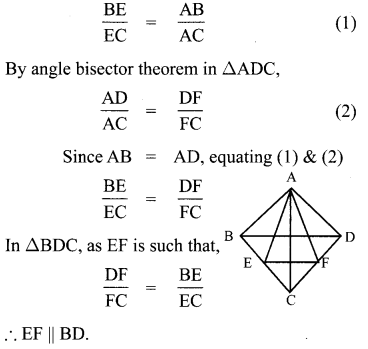Question 12.
Construct a ∆PQR which the base PQ = 4.5 cm, ∠R=35° and the median from R to RG is 6 cm.
Solution:
Construction:
Step (1) Draw a line segment PQ = 4.5 cm
Step (2) At P, draw PE such that ∠QPE = 35°.
Step (3) At P, draw PF such that ∠EPF = 90°.
Step (4) Draw ⊥r bisector to PQ which intersects PF at O.
Step (5) With O centre OP as raidus draw a circle.
Step (6) From G mark arcs of 6 cm on the circle.
Mark them as R and S.
Step (7) Join PR and RQ.
Step (8) PQR is the required triangle.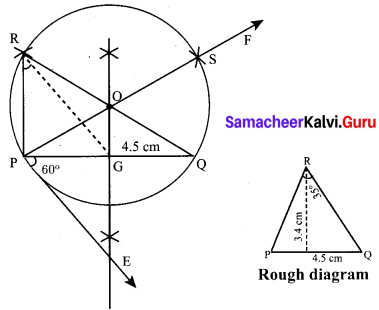Question 13.
Construct a ∆PQR in which QR = 5 cm, P = 40° and the median PG from P to QR is 4.4 cm. Find the length of the altitude from P to QR.
Solution:
Construction:
Step (1) Draw a line segment QR = 5 cm.
Step (2) At Q, draw QE such that ∠RQE = 40°.
Step (3) At Q, draw QF such that ∠EQF = 90°.
Step (4) Draw perpendicular bisector to QR, which intersects QF at O.
Step (5) With O as centre and OQ as raidus, draw a circle.
Step (6) From G mark arcs of radius 4.4 cm on the circle. Mark them as P and P’.
Step (7) Join PQ and PR.
Step (8) PQR is the required triangle.
Step(9) From P draw a line PN which is ⊥r to LR. LR meets PN at M.
Step (10) The length of the altitude is PM = 2.2 cm.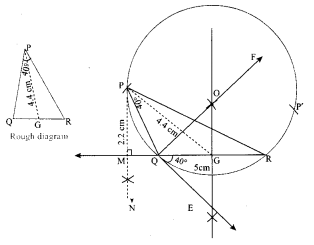Question 14.
Construct a ∆PQR such that QR = 6.5 cm, ∠P = 60° and the altitude from P to QR is of length 4.5 cm.
Solution:Construction:
Steps (1) Draw QR = 6.5 cm.
Steps (2) Draw ∠RQE = 60°.
Steps (3) Draw ∠FQE = 90°.
Steps (4) Draw ⊥r bisector to QR.
Steps (5) The ⊥r bisector meets QF at O.
Steps (6) Draw a circle with O as centre and OQ as raidus.
Steps (7) Mark an arc of 4.5 cm from G on the ⊥r bisector. Such that it meets LM at N.
Steps (8) Draw PP’ || QR through N.
Steps (9) It meets the circle at P, P’.
Steps (10) Join PQ and PR.
Steps (11) ∆PQR is the required triangle.Question 15.
Construct a ∆ABC such that AB = 5.5 cm, C = 25° and the altitude from C to AB is 4 cm.
Solution: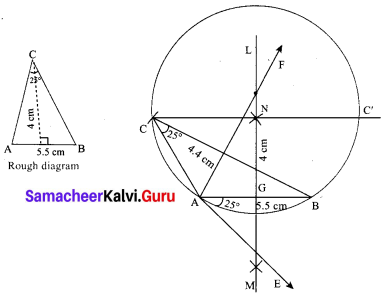Construction:
Step (1) Draw $$\overline{\mathrm{AB}}$$ = 5.5 cm
Step (2) Draw ∠BAE = 25°
Step (3) Draw ∠FAE = 90°
Step (4) Draw ⊥r bisector to AB.
Step (5) The ⊥r bisector meets AF at O.
Step (6) Draw a circle with O as centre and OA as radius.
Step (7) Mark an arc of length 4 cm from G on the ⊥r bisector and name as N.
Step (8) Draw CC1 || AB through N.
Step (9) Join AC & BC.
Step (10) ∆ABC is the required triangle.

Question 16.
Draw a triangle ABC of base BC = 5.6 cm, ∠A=40° and the bisector of ∠A meets BC at D such that CD = 4 cm.
Solution:
Construction:
Steps (1) Draw a line segment BC = 5.6 cm.
Steps (2) At B, draw BE such that ∠CBE = 60°.
Steps (3) At B draw BF such that ∠EBF = 90°.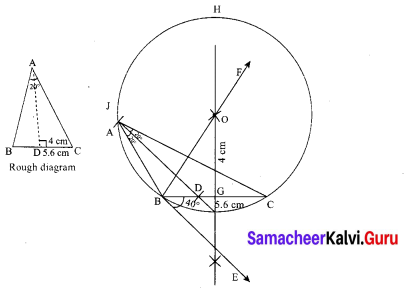Steps (4) Draw ⊥r bisector to BC, which intersects BF at 0.
Steps (5) With O as centre and OB as radius draw a circle.
Steps (6) From C, mark an arc of 4 cm on BC at D.
Steps (7) The ⊥r bisector intersects the circle at I. Join ID.
Steps (8) ID produced meets the circle at A.
Now join AB and AC. ∆ABC is the required triangle.Question 1 7.
Draw ∆PQR such that PQ = 6.8 cm, vertical angle is 50° and the bisector of the vertical angle meets the base at D where PD = 5.2 cm.
Solution: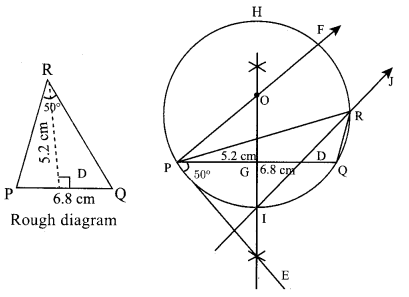Steps (1) Draw a line segment PQ = 6.8 cm
Steps (2) At P, draw PE such that ∠QPE = 50°.
Steps (3) At P, draw PF such that ∠FPE = 90°.
Step (4) Draw ⊥r bisector to PQ, which intersects PF at 0.
Step (5) With O as centre and OP as radius draw a circle.
Step (6) From P mark an arc of 5.2 cm on PQ at D.
Step (7) The ⊥r bisector intersects the circle at I. Join ID.
Step (8) ID produced meets the circle at R. Now join PR & QR. ∆PQR is the required triangle.